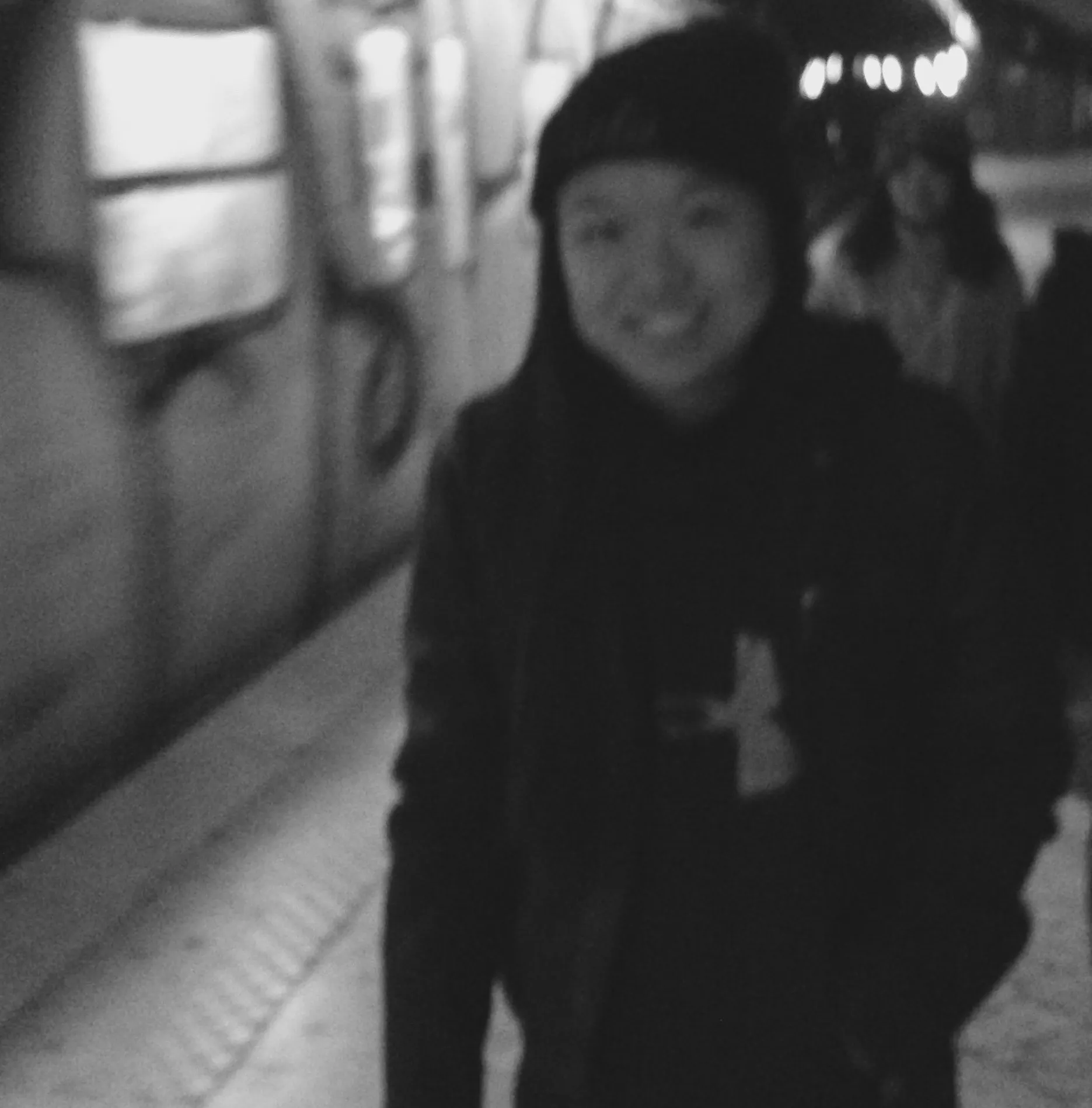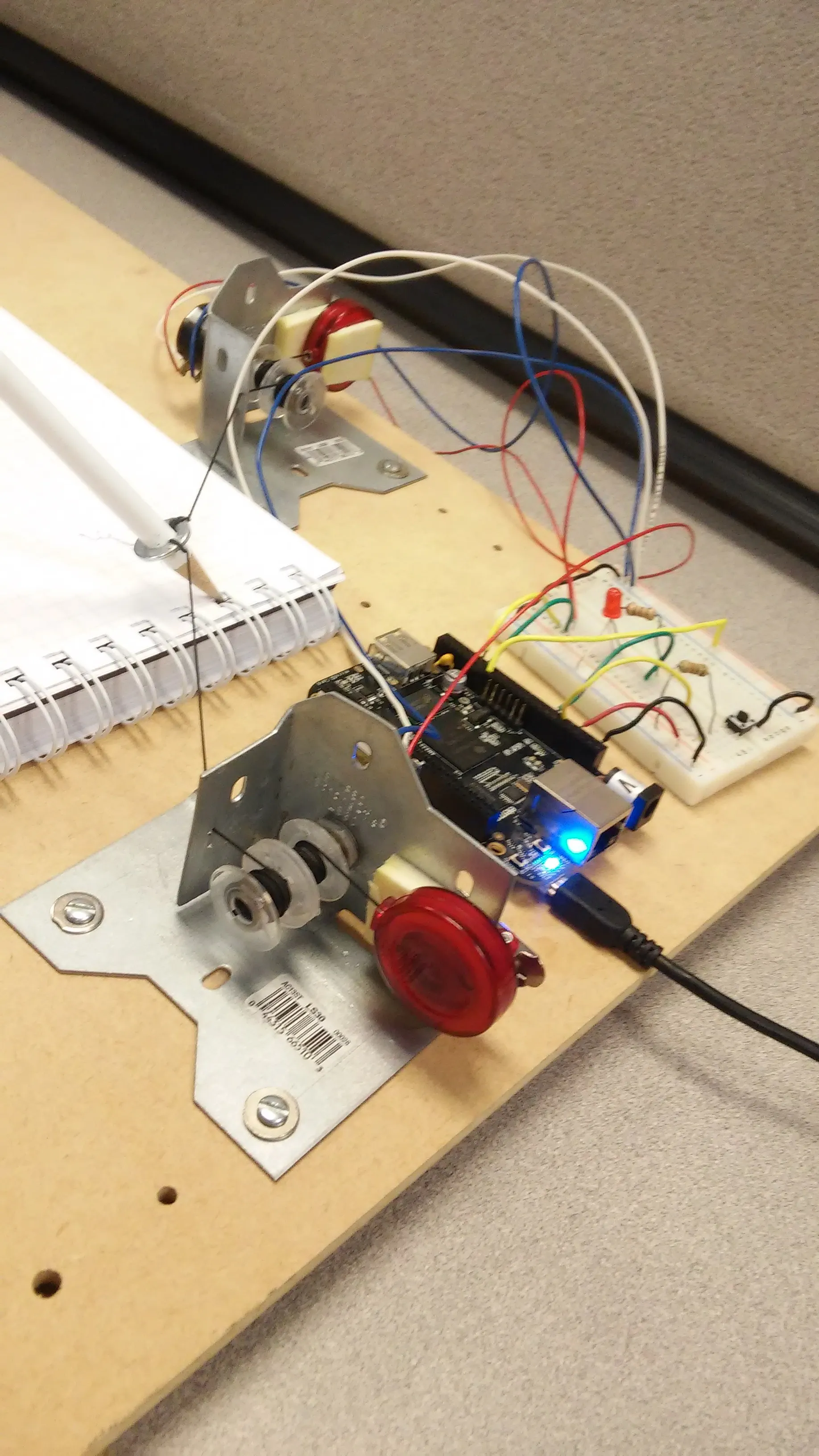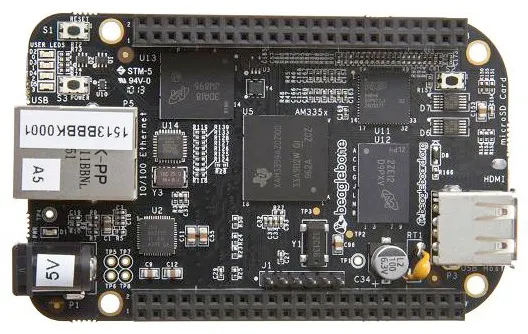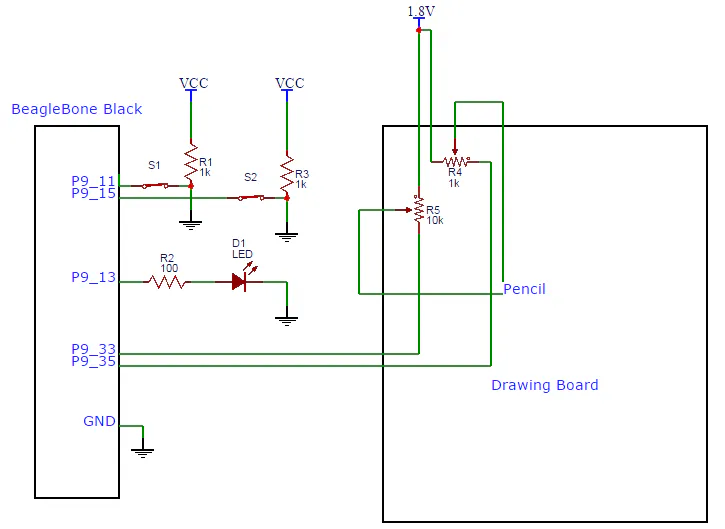Published

# Back to the Drawing Board!

Plug in the board, connect any drawing utensil of your choice, draw paper of your choice, and save your masterpiece digitally!

IntermediateFull instructions provided1,744## Things used in this project

### Hardware components

 10-turn linear potentiometer (10k)
×2
 Momentary switch
×2LED (generic)
×1BeagleBoard.org BeagleBone Black
×1
 Structural bracket
×2
×2
 Spool
×4Resistor 1k ohm
×2
 100 kΩ Resistor
×1

### Hand tools and fabrication machines

 Drill press
 Jigsaw
 Hand drill
 Vice
 File

## Schematics

### Back to the Drawing Board Schematic## Code

### Main Code

JavaScript
This file contains the logic for getting values for the pot and displaying it on the screen. Bonescript and Processing are required for it to run with the BBB.
```<html>
<script src = "processing.js"></script>
<script src = "bonescript.js"></script>
<body>

<canvas id="sketch"></canvas>

<script id = "script1" type  = "text/javascript">
(function() {

var canvas = document.getElementById('sketch');
var pjs = new Processing(canvas);

var LED = "P9_15";
var BUTTON = "P9_11";
var COLORBUTTON = "P9_13";
var X_POT = "P9_33";
var Y_POT = "P9_35";

//set up bonescript
function run()
{
var b = require('bonescript');
b.pinMode(BUTTON, b.INPUT);
b.pinMode(COLORBUTTON, b.INPUT);
b.pinMode(LED, b.OUTPUT);
b.pinMode(X_POT, b.INPUT);
b.pinMode(Y_POT, b.INPUT);
b.digitalWrite(LED, b.LOW);

//canvas setup
var drawPoint = false;
var black = pjs.color(0,0,0);
var red = pjs.color(255,0,0);
var green = pjs.color(0,255,0);
var blue = pjs.color(0,0,255);
var currentColor = black;
var COLORS = new Array(blue, green,red, black);
var colorIndex = 0;
var iterations = 0;

//board and drawing functionality setup
var drawMode = false;
var startNewLine = true;
var xcoord = 0;
var ycoord = 0;
var prev_xcoord = 0;
var prev_ycoord = 0;
var xmin = 0.15;
var ymin = 0.15;
var xmax = 0.95;
var ymax = 0.95;
var xraw = 0;
var yraw = 0;
var board_width = 0;
var board_height = 0;
var canvas_width = 512;
var canvas_height = 512;

pjs.setup = function()
{

pjs.size(canvas_width,canvas_height);
pjs.background(240);
pjs.fill(black);
pjs.rect(450,450,62,62);
pjs.fill(red);
pjs.rect(450,388,62,62);
pjs.fill(green);
pjs.rect(450,326,62,62);
pjs.fill(blue);
pjs.rect(450,264,62,62);

};

pjs.draw = function()
{

if (pjs.keyPressed==true)
{
if (pjs.key=='c' || pjs.key=='C')
{
pjs.setup();
}
}

if (iterations >20)
{
iterations = 0;
}
else{
iterations++;
}

if (drawMode==true && xcoord < 450)
{
pjs.strokeWeight(2);
pjs.stroke(currentColor);
pjs.beginShape(pjs.LINES);
pjs.vertex(prev_xcoord, prev_ycoord);
pjs.vertex(xcoord, ycoord);
prev_xcoord = xcoord;
prev_ycoord = ycoord;
pjs.endShape();

}
}

function onColorSwitch(y)
{
console.log(colorIndex)
if(y.value==b.LOW)
{
console.log("low")
if(colorIndex == COLORS.length-1)
{
colorIndex = 0;
}
else
{
console.log("in increment")
colorIndex ++;
}
switch (colorIndex)
{
case 0:
console.log("in 0")

currentColor = COLORS;
break;
case 1:
console.log("in 1")

currentColor=COLORS;

break;
case 2:
console.log("in 2")

currentColor = COLORS;
break;
case 3:
console.log("in 3")

currentColor = COLORS;
break;
}
}

}

//determine how to handle button HIGH vs button LOW
function onButtonPress(x)
{
var temp = voltageToCoords()
if (x.value==b.LOW)
{
b.digitalWrite(LED, b.HIGH);  //turn on indicator LED

if (temp ==false)
{
drawMode = false;
return;
}
if (startNewLine==true)
{
//sleep(200);
prev_xcoord = xcoord;
prev_ycoord = ycoord;
startNewLine = false;       //currently drawing this line, so set to false
}

//select a color
if (xcoord > 450 && xcoord < 512 && ycoord > 264 && ycoord < 326)
currentColor = blue;
if (xcoord > 450 && xcoord < 512 && ycoord > 326 && ycoord < 388)
currentColor = green;
if (xcoord > 450 && xcoord < 512 && ycoord > 388 && ycoord < 450)
currentColor = red;
if (xcoord > 450 && xcoord < 512 && ycoord > 450 && ycoord < 512)
currentColor = black;
drawMode = true;
}
else
{
drawMode = false;           //turn off drawing mode
b.digitalWrite(LED, b.LOW); //turn off indicator LED
startNewLine = true;        //get ready to start new line
}
}

function voltageToCoords()
{
{
xraw = x.value;
}
{
yraw = y.value;
}
xcoord = ((xraw - xmin) / (xmax - xmin)) * canvas_width;
ycoord = ((yraw - ymin) / (ymax - ymin)) * canvas_height;

if (xcoord < 0 || ycoord < 0)
return false;
}
function sleep(milliseconds)
{
var start = new Date().getTime();
for (var i=0; i<1e7; i++)
{
if ((new Date().getTime() - start) > milliseconds)
break;
}
}

pjs.setup();
pjs.draw();
pjs.loop();

}
})();

</script>
</body>
</html>
```

## Credits

### Priscilla Cheng

0 projects • 3 followers

### Tony Abdo

0 projects • 3 followers

### Rahul Ramakrishnan

0 projects • 2 followers